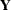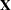MODEL Statement
MODEL response-variables = predictor-effects </ options> ;

The MODEL statement names the responses and the predictors, which determine theandmatrices of the model, respectively. Usually you simply list the names of the predictor variables as the model effects, but you can also use the effects notation of PROC GLM to specify polynomial effects and interactions; see the section Specification of Effects in Chapter 41, The GLM Procedure for further details. The MODEL statement is required. You can specify only one MODEL statement (in contrast to the REG procedure, for example, which allows several MODEL statements in the same PROC REG run).

You can specify the following options in the MODEL statement after a slash (/).

INTERCEPT

By default, the responses and predictors are centered; thus, no intercept is required in the model. You can specify the INTERCEPT option to override the default.

SOLUTION

lists the coefficients of the final predictive model for the responses. The coefficients for predicting the centered and scaled responses based on the centered and scaled predictors are displayed, as well as the coefficients for predicting the raw responses based on the raw predictors.